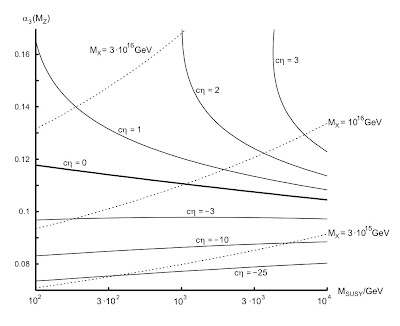## Sunday, May 04, 2008

### Grand unification and quantum gravitational effects

New paper! http://arxiv.org/abs/0805.0145

Ever notice that the energy scale for grand unification (> 10^16 GeV, as dictated by proton decay) is uncomfortably close to the scale where (currently uncalculable) quantum gravity effects are strong (Planck scale = 10^19 GeV)? Does this make you nervous about whether one can trust low-energy predictions of grand unified models? Or, conversely, whether evolution of coupling constants from low-energies can really offer evidence for or against unification?

In this new paper, we add an additional wrinkle: the scale of quantum gravity might be lower than previously expected, due to renormalization effects. In models with large numbers of particle species (e.g., N=1000, common in many SO(10), E8xE8 and even SU(5) models), these effects can reduce the Planck scale from the conventional value (10^19 GeV, obtained by dimensional analysis) by almost an order of magnitude, thereby increasing the size of quantum gravity uncertainties.

Grand unification and enhanced quantum gravitational effects

Xavier Calmet, Stephen D.H. Hsu, David Reeb

In grand unified theories with large numbers of fields, renormalization effects significantly modify the scale at which quantum gravity becomes strong. This in turn can modify the boundary conditions for coupling constant unification, if higher dimensional operators induced by gravity are taken into consideration. We show that the generic size of these effects from gravity can be larger than the two-loop corrections typically considered in renormalization group analyses of unification. In some cases, gravitational effects of modest size can render unification impossible.Figure caption: For $\eta$ fixed by the particle content of the theory, solid lines are contours of constant $c$ such that, under the presence of the gravitationally induced and enhanced operator (\ref{dim5}), SUSY-SU(5) perfectly unifies at two loops for given values of the initial strong coupling constant $\alpha_3(M_Z)$ and SUSY breaking scale $M_{{\rm SUSY}}$. Over the whole range, unification happens for some value of the coefficient $c$, with unification scale and unified coupling between $M_X=9.3\times 10^{14}\,{\rm GeV}$, $\alpha_G=0.033$ (lower right corner) and $M_X=5.5\times 10^{16}\,{\rm GeV}$, $\alpha_G=0.045$ (upper left).